• 循环队列的一些基本操作 void InitQueue(SqQueue &Q) { // 构造一个队列Q Q.base = (QElemType *)malloc(MAX_QSIZE*sizeof(QElemType)); if (!Q.base) // 存储分配失败 exit(OVERFLOW); Q.front = Q....
循环队列的一些基本操作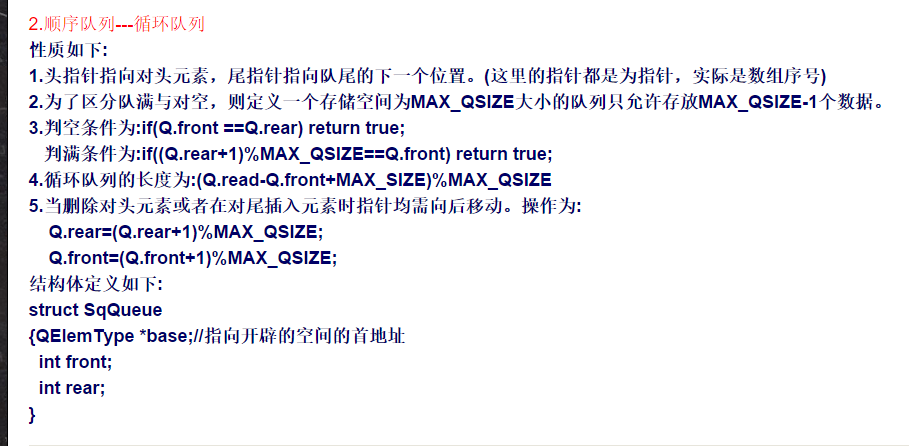void InitQueue(SqQueue &Q)
{ // 构造一个空队列Q
Q.base = (QElemType *)malloc(MAX_QSIZE*sizeof(QElemType));
if (!Q.base) // 存储分配失败
exit(OVERFLOW);
Q.front = Q.rear = 0;
}

void DestroyQueue(SqQueue &Q)
{ // 销毁队列Q，Q不再存在
if (Q.base)
free(Q.base);
Q.base = NULL;
Q.front = Q.rear = 0;
}

void ClearQueue(SqQueue &Q)
{ // 将Q清为空队列
Q.front = Q.rear = 0;
}

Status QueueEmpty(SqQueue Q)
{ // 若队列Q为空队列，则返回TRUE；否则返回FALSE
if (Q.front == Q.rear) // 队列空的标志
return TRUE;
else
return FALSE;
}

int QueueLength(SqQueue Q)
{ // 返回Q的元素个数，即队列的长度
return(Q.rear - Q.front + MAX_QSIZE) % MAX_QSIZE;
}

{ // 若队列不空，则用e返回Q的队头元素，并返回OK；否则返回ERROR
if (Q.front == Q.rear) // 队列空
return ERROR;
e = Q.base[Q.front];//等价于e=*(Q.base+Q.front)
return OK;
}

Status EnQueue(SqQueue &Q, QElemType e)
{ // 插入元素e为Q的新的队尾元素
if ((Q.rear + 1) % MAX_QSIZE == Q.front) // 队列满
return ERROR;
Q.base[Q.rear] = e;//等价于*(Q.base+Q.rear)=e
Q.rear = (Q.rear + 1) % MAX_QSIZE;
return OK;
}

Status DeQueue(SqQueue &Q, QElemType &e)
{ // 若队列不空，则删除Q的队头元素，用e返回其值，并返回OK；否则返回ERROR
if (Q.front == Q.rear) // 队列空
return ERROR;
e = Q.base[Q.front];
Q.front = (Q.front + 1) % MAX_QSIZE;
return OK;
}

void QueueTraverse(SqQueue Q, void(*vi)(QElemType))
{ // 从队头到队尾依次对队列Q中每个元素调用函数vi()
int i;
i = Q.front;
while (i != Q.rear)
{
vi(Q.base[i]);
i = (i + 1) % MAX_QSIZE;
}
printf("\n");
}
详细课件严慧敏数据结构64页
头指针主要用于删除元素的时候，删除时，元素位置向后移，尾指针主要用于在添加元素的时候，添加元素时，尾指针向后移，
一般队列的判空
front == rear
一般队列的判满
为 rear -front ==MAX_QUEUE_SIZE
<STRONG><SPAN style="COLOR: #000066; FONT-SIZE: 18px">void InitQueue(LinkQueue &Q)
{ // 构造一个空队列Q
if(!(Q.front=Q.rear=(QueuePtr)malloc(sizeof(QNode))))
exit(OVERFLOW);
Q.front->next=NULL;
}

{ // 销毁队列Q(无论空否均可)
while(Q.front)
{
Q.rear=Q.front->next;
free(Q.front);
Q.front=Q.rear;//释放一块内存要做两点：1.释放指向它的指针。2.将该指针指向空
}
}

{ // 将Q清为空队列
QueuePtr p,q;
Q.rear=Q.front;
p=Q.front->next;
Q.front->next=NULL;//只留下头结点
while(p)
{
q=p;
p=p->next;
free(q);
}
}

{ // 若Q为空队列，则返回TRUE，否则返回FALSE
if(Q.front->next==NULL)//注意不要把链式队列的判空条件与循环队列混淆
return TRUE;
else
return FALSE;
}

{ // 求队列的长度
int i=0;
QueuePtr p;
p=Q.front;
while(Q.rear!=p)
{
i++;
p=p->next;
}
return i;
}

{ // 若队列不空，则用e返回Q的队头元素，并返回OK，否则返回ERROR
QueuePtr p;
if(Q.front==Q.rear)
return ERROR;
p=Q.front->next;
e=p->data;
return OK;
}

{ // 插入元素e为Q的新的队尾元素
QueuePtr p;
if(!(p=(QueuePtr)malloc(sizeof(QNode)))) // 存储分配失败
exit(OVERFLOW);
p->data=e;
p->next=NULL;
Q.rear->next=p;
Q.rear=p;
}

{ // 若队列不空，删除Q的队头元素，用e返回其值，并返回OK，否则返回ERROR
QueuePtr p;
if(Q.front==Q.rear)
return ERROR;
p=Q.front->next;
e=p->data;
Q.front->next=p->next;
if(Q.rear==p)
Q.rear=Q.front;//如果只有一个节点，那么删除这个节点后rear指针也就丢了，需重新赋值
free(p);
return OK;
}

{ // 从队头到队尾依次对队列Q中每个元素调用函数vi()
QueuePtr p;
p=Q.front->next;
while(p)
{
vi(p->data);
p=p->next;
}
printf("\n");
}</SPAN></STRONG>
[cpp] view plaincopyprint?
{ // 构造一个空队列Q
if(!(Q.front=Q.rear=(QueuePtr)malloc(sizeof(QNode))))
exit(OVERFLOW);
Q.front->next=NULL;
}

{ // 销毁队列Q(无论空否均可)
while(Q.front)
{
Q.rear=Q.front->next;
free(Q.front);
Q.front=Q.rear;//释放一块内存要做两点：1.释放指向它的指针。2.将该指针指向空
}
}

{ // 将Q清为空队列
QueuePtr p,q;
Q.rear=Q.front;
p=Q.front->next;
Q.front->next=NULL;//只留下头结点
while(p)
{
q=p;
p=p->next;
free(q);
}
}

{ // 若Q为空队列，则返回TRUE，否则返回FALSE
if(Q.front->next==NULL)//注意不要把链式队列的判空条件与循环队列混淆
return TRUE;
else
return FALSE;
}

{ // 求队列的长度
int i=0;
QueuePtr p;
p=Q.front;
while(Q.rear!=p)
{
i++;
p=p->next;
}
return i;
}

{ // 若队列不空，则用e返回Q的队头元素，并返回OK，否则返回ERROR
QueuePtr p;
if(Q.front==Q.rear)
return ERROR;
p=Q.front->next;
e=p->data;
return OK;
}

{ // 插入元素e为Q的新的队尾元素
QueuePtr p;
if(!(p=(QueuePtr)malloc(sizeof(QNode)))) // 存储分配失败
exit(OVERFLOW);
p->data=e;
p->next=NULL;
Q.rear->next=p;
Q.rear=p;
}

{ // 若队列不空，删除Q的队头元素，用e返回其值，并返回OK，否则返回ERROR
QueuePtr p;
if(Q.front==Q.rear)
return ERROR;
p=Q.front->next;
e=p->data;
Q.front->next=p->next;
if(Q.rear==p)
Q.rear=Q.front;//如果只有一个节点，那么删除这个节点后rear指针也就丢了，需重新赋值
free(p);
return OK;
}

{ // 从队头到队尾依次对队列Q中每个元素调用函数vi()
QueuePtr p;
p=Q.front->next;
while(p)
{
vi(p->data);
p=p->next;
}
printf("\n");
}</span></strong>

详细可见
http://blog.csdn.net/kkkkkxiaofei/article/details/8482066这个博客

展开全文• ☝☝☝软件工程考研独家平台撰稿| 康康哥编辑| 丽丽姐本文由懂计算机、软件工程的博士师哥原创双日练：NO.20200922循环队列放在一维数组A[0…M-1]中，end1指向队头元素，end2指向队尾元素的后一个位置。假设队列两端...


☝☝☝ 软件工程考研独家平台撰稿 | 康康哥编辑 | 丽丽姐本文由懂计算机、软件工程的博士师哥原创双日练：NO.20200922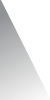循环队列放在一维数组A[0…M-1]中，end1指向队头元素，end2指向队尾元素的后一个位置。假设队列两端均可进行入队和出队操作，队列中最多能容纳M-1个元素。初始时为空。下列判断队空和队满的条件中，正确的是 ( )。A．队空：end1 == end2；队满：end1 == (end2+1)mod MB．队空：end1 == end2；队满：end2 == (end1+1)mod (M-1)C．队空：end2 == (end1+1)mod M；队满：end1 == (end2+1)mod MD.  队空：end1 == (end2+1)mod M；队满：end2 == (end1+1)mod (M-1)本题考查：循环队列判断队空队满。循环队列判断队满：Q.front== (Q.rear + 1) % MAXSIZE；判断队空： Q.front== Q.rear；将end1替换front，end2替换rear，因此得：队空：end1 == end2；队满：end1 == (end2+1)modM。因此选A。软工博士带你飞考软工 · 看CS优化狮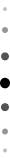展开全文• 一、说明 循环队列就是将队列存储空间的最后一个位置绕到第一个位置，形成逻辑上的环状...因此，队列判空条件是front=rear，而队列判满的条件是front=（rear+1)%MaxSize。 二、代码 #ifndef CYCLEQUEUE_H #define C
一、说明
循环队列就是将队列存储空间的最后一个位置绕到第一个位置，形成逻辑上的环状空间，供队列循环使用。在循环队列中，当队列为空时，有front=rear，而当所有队列空间全占满时，也有front=rear。为了区别这两种情况，规定循环队列最多只能有MaxSize-1个队列元素，当循环队列中只剩下一个空存储单元时，队列就已经满了。因此，队列判空的条件是front=rear，而队列判满的条件是front=（rear+1)%MaxSize。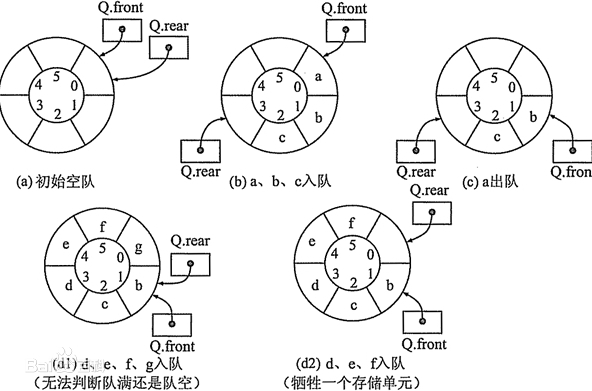二、代码
#ifndef CYCLEQUEUE_H
#define CYCLEQUEUE_H
#include <iostream>
#include <vector>
#define MAXSIZE 6 //最大队列长度
using namespace std;
class cycleQueue
{
public:
cycleQueue();
int length();
bool insert(int x);
bool del();
void test();
void print();
private:
int* m_pBase;
int m_front;//头指针，若队列不空，指向队列头元素
int m_rear;//尾指针，若队列不空，指向队列尾元素的下一个位置
};

#endif // CYCLEQUEUE_H


#include "cyclequeue.h"

cycleQueue::cycleQueue()
:m_pBase(nullptr)
,m_front(0)
,m_rear(0)
{
m_pBase = (int*) malloc (MAXSIZE*sizeof(int));
if(!m_pBase){
cout<<"malloc fail"<<endl;
}
}

int cycleQueue::length()
{
return (m_rear - m_front + MAXSIZE) % MAXSIZE;
}

bool cycleQueue::insert(int x)
{
if((m_rear+1)%MAXSIZE == m_front){
cout<<"queue is full"<<endl;
return false;
}
m_pBase[m_rear] = x;
m_rear = (m_rear+1) % MAXSIZE;
return true;
}

bool cycleQueue::del()
{
if(m_front == m_rear){
cout<<"queue is empty"<<endl;
return false;
}
m_front = (m_front+1) % MAXSIZE;
return true;
}

void cycleQueue::test()
{
for(int i=0;i<5;i++){
insert(i);
}
print();
del();
del();
print();
insert(3);
insert(4);
insert(5);
insert(6);
print();
}
void cycleQueue::print()
{
for(int i=0;i<MAXSIZE-1;i++){
cout<<"m_pBase["<<i<<"]:"<<m_pBase[i]<<endl;
}
cout<<"m_front:"<<m_front<<endl;
cout<<"m_rear:"<<m_rear<<endl;
}




展开全文• 循环队列，也叫“环形队列”。 它指的是：将队列抽象成一个环形，如果队头前面有空间，我们...因此，环形队列判空条件是front==rear,判满的条件是(rear+1)%n==front.n表示数组总大小（n=k+1,k表示有效元素个数）...
循环队列，也叫“环形队列”。

它指的是：将队列抽象成一个环形，如果队头前面有空间，我们可以继续使用。环形队列可以用数组、链表实现，以下我们使用数组实现。环形队列的队头front表示第一个入队的元素下标，队尾rear代表最后一个有效元素的下一个位置下标。因此，环形队列判空条件是front==rear,判满的条件是(rear+1)%n==front.n表示数组总大小（n=k+1,k表示有效元素个数）。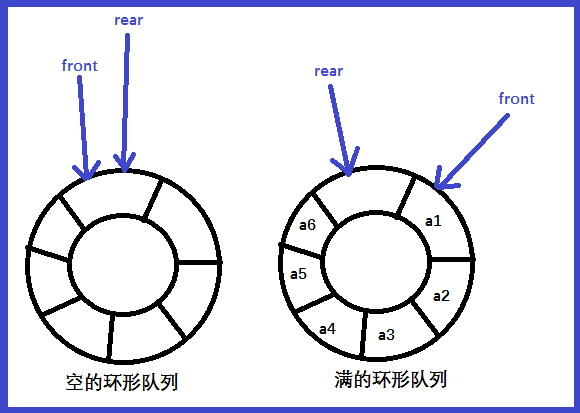以下是环形队列的设计，以及一些接口的实现：

typedef struct
{
int* queue;
int front;
int rear;
int k;//队列长度

}MyCircleQueue;

void* myCircleQueueCreate(MyCircleQueue* q, int k)//初始化
{
assert(q);
q->queue = (int*)malloc(sizeof(int)*(k+1));//注意要多开一个大小，队列长度为k指的是有效元素个数，而rear始终指向的是一个空的位置，所以这个多余的空间是给它留的
q->k = k;
q->front = q->rear = 0;
}

int myCircleQueuePush(MyCircleQueue* q, int x)//插入,返回0说明插入失败,返回1说明插入成功
{
assert(q);
if ((q->rear + 1) % (q->k + 1) == q->front)//满了
{
return 0;
}
//来到这说明未满,直接插入
q->queue[q->rear++] = x;
if (q->rear == q->k + 1)//最后一个位置的下一个位置应该是0，让其循环起来
q->rear = 0;
return 1;
}

int myCircleQueuePop(MyCircleQueue* q)//删除返回0说明插入失败,返回1说明插入成功
{
assert(q);
if (q->front == q->rear)//空
{
return 0;
}
//来到这，说明非空，直接删除
q->front++;
if (q->front == q->k + 1)
q->front = 0;//与上一个题解释相同，让其循环起来
return 1;
}

int myCircleQueueFront(MyCircleQueue* q)//取队头元素，队列空返回-1
{
assert(q);
if (q->front == q->rear)//队空
{
return -1;
}
else
{
return q->queue[q->front];
}
}

int myCircleQueueRear(MyCircleQueue* q)//取队尾元素，队列空返回-1
{
assert(q);
if (q->front == q->rear)//队空
{
return -1;
}
//来到这，说明不空,不空的情况下，如果队尾==0,说明真正有效的元素是前一个
if (q->rear == 0)
{
return q->queue[q->k];
}
else
{
return q->queue[q->rear - 1];
}
}

int myCircleQueueEmpty(MyCircleQueue* q)//判空,空返回0，非空返回1
{
assert(q);
if (q->front == q->rear)
{
return 0;
}
else
{
return 1;
}
}

int myCircleQueueFull(MyCircleQueue* q)//判满，满返回0，不满返回1
{
assert(q);
if ((q->rear + 1) % (q->k + 1) == q->front)
{
return 0;
}
else
{
return 1;
}
}

void myCircleQueueFree(MyCircleQueue* q)//销毁
{
assert(q);
free(q->queue);
free(q);
}


展开全文• 队列： 1、在一端插入在另一端删除 2、先进先出 （First In First Out，FIFO） 3、允许插入的一端为队尾，允许删除的一端为队头循环队列： 对于普通的顺序队列判空条件是front是否与rear相等且等于0，但出对时...数据结构
• 循环队列判空条件为：Q.rear == Q.fornt; 循环队列的判读队满的条件是：(Q.rear + 1) % MaxSize == Q.fornt; 下面是队列实现的存储类型结构体： #define MaxSize 50 typedef int ElemType; typedef struct { ...
• 但是在判断循环队列空和满这两种状态任然存在问题，因为对于一个循环队列，不做任何判空和判满的机制。判空和判满的条件都是：q->rear == q->front。带来的问题就是当出现上述条件时不能区分循环队列到底是空...
• //运行环境keil c51 //学习目的：循环队列的运用 /*关键算法： 在循环队列中，当队列为空...列判空条件时front=rear，而队列判满的条件时front=（rear+1）%MaxSize。 */ #include "reg51.h" #include <stdio.h>.
• 在实现循环队列之前，我来说一说其中关键的地方： 1.front和rear指针的指向 问题：rear指最后一个节点，front的指针一般来说，指向队列中第一个节点...4.判空条件：front==rear，对于这一个的记忆，你可以想象一个含
• 实验5、链队列的基本操作 实验要求： （1）实验目的 通过该实验，使学生理解链队列的构造特点并灵活应用，掌握链队基本操作的编程实现，... 队列判空，5.求队列长度，6.获取队头元素，7.插入一个 元素，8.删除一个元素数据结构 编程语言 c++
• // 判空条件：front=rear=0,判满条件：（Q.rear+1)% MaxSize==Q.front // 入栈：base[rear++]=e; 出栈：e=base[front++]; #include"pch.h" #include<iostream> #define MaxSize 200//循环队列最大长度 using ...
• 可以看看这篇文章：静态队列为什么必须是循环队列2、循环队列要点 判空队列为空的条件：head == tail 判断队列已满的条件： (head + 1) % 数组长度 == tail 入队后维护tail: tail = (tail + 1） % 数组长度 出...
• 1. 初始化 数组 头尾节点 2. 插入 ...需要预留一个位置，用于判断队列是否满了 ...5. 判空 判断队列空的条件： rear==front; 打印整个队列： for循环所需条件： 开始下标：front 有效个数：(rear+maxSize-数据结构 算法
• 循环队列判空，判满条件要综合考虑（可以推导出来） 遍历的时候，需要是由到当前的size，然后通过取模操作取出循环队列中的值 code package com.wrial.queue; /* * @Author Wrial * @Date Created in 15:40 ...
• 同时，由于环状的特殊，判满和判空条件需要区分，故在空间中额外留出一个位置个尾指针，尾指针为最后一个元素的下一个空间，这样当(rear==front)时即为空队列，当(rear+1)%max_size == front时即为队满（想象环形...
• 循环队列节约了一定的空间，仍然用数组实现。 要注意的是 rear是队尾元素下一个单元的下标 front == rear时队列为 人为地让一个存储单元不存储任何信息，条件为 （rear+1+MAXSIZE)%MAXSIZE == front 之所以...
• （2） 分别定义循环队列的基本条件（初始化队列、、入队、出队等） （3） 设计一个测试主函数进行测试 三：实验要求 根据内容编写程序，上机调试并获得运行结果 四：程序清单、调试和测试结果及分析 #...数据结构 c语言
• 数组模拟队列1.顺序队列2.循环队列 1.顺序队列 指针位置 front：指向队列的第一...判空条件if (front == rear) { return true; } 队列满判断条件if (rear == maxSize - 1) { return true; } 有效队列长度size =java
• 第三章 栈和队列;学习提要 1....熟练掌握循环队列和链队列的基本操作实现 算法特别注意队满和队空的描述方法 重难点内容 顺序栈的相关操作循环队列判空判满;3.1 栈stack;栈的定义和特点 定义限定仅
• 答：有以下四种方法判定循环队列的空满（假设头为front尾为rear，循环队列的最大为Maxsize）预留一位：可以空余出来一个存储单元，不储存任何数，则判空条件是front%Maxsize == rear%Maxsize 判满条件是(front+1)%...
• 在顺序栈中,若栈顶指针top指向栈顶元素的下一个存储单元,且顺序栈的最大容量是maxSize,则顺序栈的判空条件是( )。top==0；【顺序栈是数组；另一种栈顶指针：将top指向栈顶元素的存储位置，空栈时top=-1】 循环顺序...
• 逻辑结构：线性结构、非线性结构 存储结构：顺序存储、链接存储、索引存储、散列存储 数据运算：插入、删除、查找...判空条件：front == rear 判满条件： front == （rear+1）%N 循环移动：r = （r+1）%N 深度优先数据结构 matrix 存储 算法
• 4.循环队列判空判满条件 5.OSI/RM模型 6.ABCD类网络地址范围 7.线程安全与线程不安全 8.GDB是Linux下的程序调试工具，主要有哪些功能 9.C++运算符的优先级 还有几道编程题（多做下剑指offer上的练习，还链表 数据结构
• BFS主要是用队列实现，具体模版是入队第一个元素，当队列不为时进行循环，如果需要记录每层的数据（层数），就保存队列的长度，在队列不为循环里进行for长度的循环，先出队第一个元素，进行条件判断，满足条件...
• 2.1课程设计内容 该课题要求以邻接表的方式存储图，输出邻接表，并要求实现图的深度、广度两种遍历。 2.1.1图的邻接表的建立与...并以队列是否为作为循环控制条件。对于深度优先遍历则采用递归或非递归算法来实现。数据结构